Home | Introduction | Software | Examples | History | People | Bibliography | Applications | Theorems

## Pseudospectra of Toeplitz Matrices and Operators: Matrices with Piecewise Continuous Symbols

Recall that the entries of a Toeplitz matrix are the Laurent coefficients of a function known as the symbol, whose domain is the unit circle. If a Toeplitz matrix is banded, then the symbol is a trigonometric polynomial, and the resolvent norm of the finite dimensional operator grows exponentially at points within the spectrum of the corresponding infinite dimensional operator. The pseudospectra of finite dimensional Toeplitz matrices converge to those of the infinite dimensional operator, and this convergence is extremely rapid (exponential in the matrix dimension). For examples, see the page of banded Toeplitz examples.

Böttcher proved that the pseudospectra of the finite dimensional Toeplitz matrices must converge to the pseudospectra of the infinite dimensional operator even when the symbol is only piecewise continuous [Böt94]. This class of functions is much broader than the trigonometric polynomials described above. Do the pseudospectra still converge rapidly to their infinite dimensional Limits when a discontinuity is present? The answer is no, in a sense made precise in [BET00]. The examples below, which should be contrasted with the banded Toeplitz examples, illustrate this slow convergence.

Our first example arises from the symbol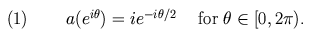The entries of the Toeplitz matrix determined by (1) are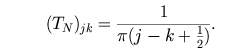The spectrum of the corresponding infinite dimensional Toeplitz operator is the right half of the closed unit disk. As seen from the plots in Figure 1, the pseudospectra are far from the unit disk even for relatively large values of epsilon and large matrix dimensions.

This example is related to the "Moler phenomenon", described in Section 4.5 of [BS99]. The very slow growth of the resolvent norm at z=0 for this example (logarithmic in the matrix dimension) was established by Tyrtyshnikov [Tyr92].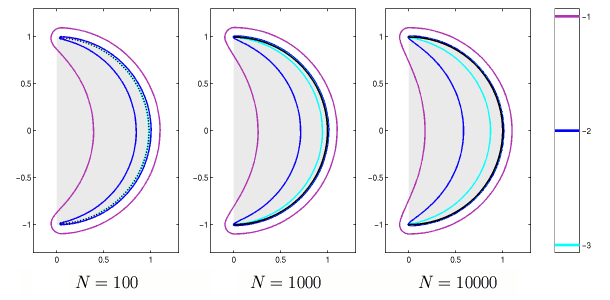Figure 1. Spectrum and epsilon-pseudospectra for Toeplitz matrices of dimensions N=100, N=1000, and N=10000 corresponding to the symbol (1). The symbol of the infinite dimensional operator is the right half of the unit disk, shaded gray. A fast Toeplitz algorithm was used to calculate the N=10000 data, and computations were performed on an SGI Cray Origin2000 at the Oxford Supercomputing Center.

Our second example shows similarly slow growth of the pseudospectra for matrices associated with the symbol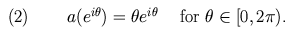The pseudospectra for three matrices defined by (2) are shown in Figure 2.

The limiting behavior of the eigenvalues of the finite dimensional Toeplitz matrices associated with (1) and (2) has been investigated by Basor and Morrison [BM94].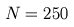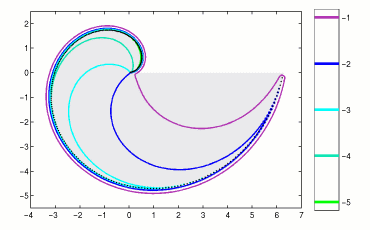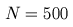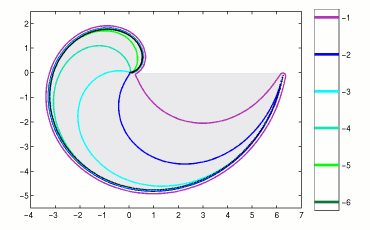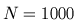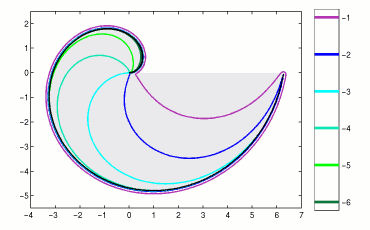Figure 2. Spectrum and epsilon-pseudospectra for Toeplitz matrices of dimensions N=250, N=500, and N=1000 corresponding to the symbol (2). The gray filled region is the spectrum of the infinite dimensional operator.

# Supplemental Bibliography

References to works included in the Pseudospectra Bibliography are presented as links above, and are not repeated here.
 [BM94] E. L. Basor and K. E. Morrison The Fisher-Hartwig conjecture and Toeplitz eigenvalues Linear Algebra Appl. 202 (1994), 129-142. MR 95g:47037
 [Tyr92] E. E. Tyrtyshnikov Singular values of Cauchy-Toeplitz matrices Linear Algebra Appl. 151 (1992), 99-116. MR 92m:47056